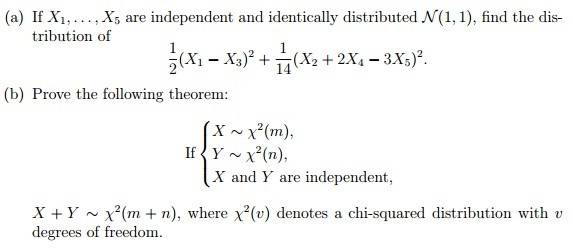# Questions about Linear Combinations of Random Variables

• ken15ken15

## Homework Statement## Homework Equations

Y=1/2*(X1-X3)^2+1/14*(X2+2X4-3X5)^2

## The Attempt at a Solution

For (a) part, I have only learned to find the moment-generating function of Y, but not finding the p.d.f.
Moreover, the examples I have seen only involves random variables Xi to the power 1, but not to higher power.

For (b) part, the difficulty for me is just similar to part (a).

Last edited:
Hint: $$(Z_1^2 + ... +Z_n^2) +(Z_1^2 + ... +Z_m^2) = (Z_1^2 + ... +Z_n^2 +...+ Z_m^2)$$

Hint: $$(Z_1^2 + ... +Z_n^2) +(Z_1^2 + ... +Z_m^2) = (Z_1^2 + ... +Z_n^2 +...+ Z_m^2)$$

sorry but...what property is it? I haven't learned this before...

## Homework Statement## Homework Equations

Y=1/2*(X1-X3)^2+1/14*(X2+2X4-3X5)^2

## The Attempt at a Solution

For (a) part, I have only learned to find the moment-generating function of Y, but not finding the p.d.f.
Moreover, the examples I have seen only involves random variables Xi to the power 1, but not to higher power.

For (b) part, the difficulty for me is just similar to part (a).

##Z_1 = X_1 - X_3## has an ##N(0,a)## distribution, ##Z_2 = X_2+2X_4-3X_5## is ##N(0,b)##, and ##Z_1, Z_2## are independent. So ##Y## involves a weighted sum of squares of independent, mean-0 random variables.

##Z_1 = X_1 - X_3## has an ##N(0,a)## distribution, ##Z_2 = X_2+2X_4-3X_5## is ##N(0,b)##, and ##Z_1, Z_2## are independent. So ##Y## involves a weighted sum of squares of independent, mean-0 random variables.

So do you mean given the random variables are independent, the sum or difference of them will also have the same distribution of them? Also, how can a duel with the square of the random variables? Do I need to consider it with transformation of variables?